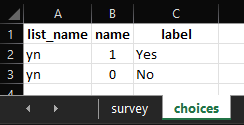# Counting number of responses from a form

Hello, I’d like to do something similar but not sure how. I have a series of questions that can be aswered 0, 60, 100 for examples. I’d like to know how many questions were anwered 0, how many were answered 60 and how many were answered 100. Is that feasible directly in the kobo form?

Thank you!

Best

Diane

Hello,

Anyone would have an idea for counting how many questions were answered 0, how many were answered 60, how many were answered 100 etc?

Thank you

@dianedetoeuf, in a survey project or in a single survey form (that was filled up)?

On a single survey form that was filled up.
When they were collecting on paper they were then using the Countif function on excel to calculate how many answers were 0, how many were 60 etc. I’m trying to do the same on the Kobo form… But so far all the count functions seem to have been used only to calculate the number of answers selected in 1 questions, not the number of answers responding to a specific criteria over multiple questions (those questions are select_ones). Does that make sense?

@dianedetoeuf, are you talking of something that should count the response as shown in the image below:

#### In the choices tab of your XLSForm:#### Reference XLSForm:

Counting Response.xlsx (10.8 KB)

Its a bit more difficult than that because my select_ones have more than 2 choices, and I also need to use results from previous calculated questions. Here is the form so you can have a look, the calculations I want to do are lines 88, 89 and 90.

Fishery_assessment_20211208.xlsx (61.9 KB)

OK @dianedetoeuf, could you list out a dummy question maybe 2-3, and also their possible choices. Then please provide the scenario of your calculation on how you wish to see. Maybe the community should be able to help you out.

Sure, here is a simplified example with what the results should look like:

And the simplified form is attached. Would be extremely grateful if this can be solved by the community !!
scoring_test.xlsx (20.9 KB)

Thanks

1 Like

This is very clear now.

@dianedetoeuf, please correct me if I am wrong. Should it be in the way as shown below:

Hi Kal,

Actually on Example result 2, because S1 and S2 scores are both 0 then the number of scores between 0 and 60 is 2, so LowRiskScore is 2.
On example 3, the 3 scores are 0, so the LowRiskScore is 3.
Does that make sense?

@dianedetoeuf, maybe this should solve your issue:

#### Reference XLSForm:

scoring_test.xlsx (20.1 KB)

Will try that, thank you so much @Kal_Lam!

1 Like